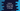# Python numpy empty_like method example## How to use empty_like in numpy with example:

empty_like is a method of NumPy that returns a new array with same shape and type as the provided array. In this post, I will show you how to use empty_like method with examples.

## Definition of empty_like:

empty_like is defined as like below:

``empty_like(p, dtype=None, order='K', subok=True, shape=None)``

Here,

• p is the prototype, the data-type and shape define the same attributes of the returning array. It is an array_like parameter.
• dtype is a data-type optional parameter. It is used to override the data type of the result array.
• order is an optional parameter that overrides the memory layout of the result array. It can be ‘C’, ‘F’, ‘A’ or ‘K’.
• subok is an optional boolean value. For False, the result array will be base-class array. If True, it will use the sub class type of prototype.
• shape is an optional value, it can be int or sequence of ints. It overrides the shape of the result array.

### Example program:

Let’s try this with an example:

``````import numpy as np

given_arr = ([1,2],[3,4], [5,6])

print(np.empty_like(given_arr))``````

It will print one output as like below:

``````[[-4611686018427387904 -4611686018427387904]
[ 4616981938510757898  4613349226564724111]
[-4611686018427387904 -4611686018427387904]]``````

It created an uninitialized array.

Each time you run this program, it will create one different uninitialized array.

### With a different dtype:

Let’s change the data type of the returned array to string:

``````import numpy as np

given_arr = ([1,2],[3,4], [5,6])

print(np.empty_like(given_arr, dtype=str))``````

It will give:

``````[['' '']
['' '']
['' '']]``````